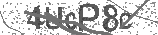﻿ How Quantum Computers Break Encryption | Shor's Algorithm Explained | Safe Videos for Kids
Welcome

# How Quantum Computers Break Encryption | Shor's Algorithm Explained

Thanks! Share it with your friends!

URL

You disliked this video. Thanks for the feedback!

Sorry, only registred users can create playlists.URLFind Related Videos  added
251 Views## Description

Support MinutePhysics on Patreon! http://www.patreon.com/minutephysics

This video explains Shors Algorithm, a way to efficiently factor large pseudoprime integers into their prime factors using a quantum computer. The quantum computation relies on the number-theoretic analysis of the factoring problem via modular arithmetic mod N (where N is the number to be factored), and finding the order or period of a random coprime number mod N. The exponential speedup comes in part from the use of the quantum fast fourier transform which achieves interference among frequencies that are not related to the period (period-finding is the goal of the QFT FFT).

REFERENCES

RSA Numbers (sample large numbers to try factoring)
https://en.wikipedia.org/wiki/RSA_numbers

IBM on RSA
https://www.ibm.com/support/knowledgecenter/en/SSB23S1.1.0.13/gtps7/s7pkey.html

Modulo Multiplication Group Tables
http://mathworld.wolfram.com/ModuloMultiplicationGroup.html

Difference of squares factorization
https://en.wikipedia.org/wiki/Difference_of_two_squares

Euclids Algorithm
https://en.wikipedia.org/wiki/Euclideanalgorithm

Rational sieve for factoring
https://en.wikipedia.org/wiki/Rational_sieve

General Number field Sieve
https://en.wikipedia.org/wiki/Generalnumberfieldsieve

Scott Aaronson blog post about Shors Algorithm
https://www.scottaaronson.com/blog/?p=208

Experimental implementation of Shors Algorithm (factoring 15, 21, and 35)
https://arxiv.org/pdf/1903.00768.pdf

Adiabatic Quantum Computation factoring the number 291311
https://arxiv.org/pdf/1706.08061.pdf

Scott Aaronson course notes
https://www.scottaaronson.com/qclec/
https://www.scottaaronson.com/qclec/combined.pdf

Shors Algorithm on Quantiki
https://www.quantiki.org/wiki/shors-factoring-algorithm

TLS And SSL use RSA encryption
https://en.wikipedia.org/wiki/TransportLayerSecurity

Dashlane security whitepaper

MinutePhysics is on twitter - @minutephysics

Minute Physics provides an energetic and entertaining view of old and new problems in physics -- all in a minute!

Created by Henry ReichBe the first to comment